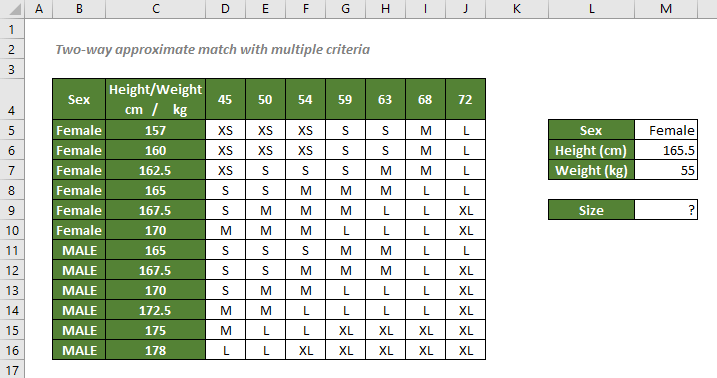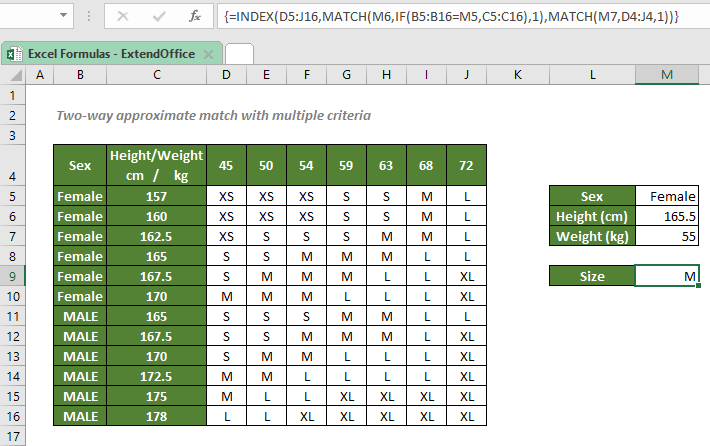## Two-way approximate match with multiple criteria

In this tutorial, we will talk about how to look for an approximate match based on multiple criteria listed both in columns and rows in an Excel spreadsheet, with the help of INDEX, MATCH, and IF functions.#### How to perform a two-way approximate match with multiple criteria in Excel?

To find out the clothing size for a woman who is 165.5cm tall and weighs 55kg according to the table above, you can add two MATCH functions to the formula: one with an IF function gets the row number (sex and height), the other gets the column number (weight). Then INDEX will find the corresponding size according to the coordinates. (Note that the row number goes ahead of the column number in an INDEX formula.)

#### Generic syntax

=INDEX(return_range,MATCH(lookup_value1,IF(lookup_array2=lookup_value2,lookup_array1),match_type),MATCH(lookup_value3,lookup_array3,match_type))

√ Note: This is an array formula that requires you to enter with Ctrl + Shift + Enter.

• return_range: The range where you want the combination formula to return the size from. Here refers to the size range.
• lookup_value: The value that the formula used to locate the position of the corresponding size. Here refers to the information about the given sex, height and weight.
• lookup_array: The range of cells with the values to compare with the lookup_value. Here refers to the sex, height and weight ranges.
• match_type: 1 or -1.
1 or omitted (default), MATCH will find the largest value that is less than or equal to the lookup_value. The values in the lookup_array must be placed in ascending order.
-1, MATCH will find the smallest value that is greater than or equal to the lookup_value. The values in the lookup_array must be placed in descending order.

To find out the clothing size for the woman who is 165.5cm tall and weighs 55kg, please copy or enter the formula below in the cell M9, and press Ctrl + Shift + Enter to get the result:

=INDEX(D5:J16,MATCH(M6,IF(B5:B16=M5,C5:C16),1),MATCH(M7,D4:J4,1))#### Explanation of the formula

=INDEX(D5:J16,MATCH(M6,IF(B5:B16=M5,C5:C16),1),MATCH(M7,D4:J4,1))

• IF(B5:B16=M5,C5:C16): The IF function checks each value in B5:B16 if they match the value in the cell M5, Female. If so, the IF function will keep the corresponding heights listed in C5:C16; If not, IF will return FALSEs. The formula will return an array like this: {157;160;162.5;165;167.5;170;FALSE;FALSE;FALSE;FALSE;FALSE;FALSE}.
• MATCH(M6,IF(B5:B16=M5,C5:C16),1) = MATCH(M6,{157;160;162.5;165;167.5;170;FALSE;FALSE;FALSE;FALSE;FALSE;FALSE},1): The match_type 1 forces the MATCH function to return the position of the largest value that is less than or equal to 165.5 (the value in the cell M6) in the array. So, MATCH will return 4, the position of the value 165.
• MATCH(M7,D4:J4,1): The MATCH function returns 3, since the largest value that is less than or equal to the value in M7, 55, is at the 3rd position of the range D4:J4.
• INDEX(D5:J16,MATCH(M6,IF(B5:B16=M5,C5:C16),1),MATCH(M7,D4:J4,1)) = INDEX(D5:J16,4,3): The INDEX function retrieves the value at the intersection of the 4th row and 3rd column in the size range D5:J16, which is the value in the cell F8, M.

#### Related functions

Excel INDEX function

The Excel INDEX function returns the displayed value based on a given position from a range or an array.

Excel MATCH function

The Excel MATCH function searches for a specific value in a range of cells, and returns the relative position of the value.

Excel IF function

The IF function is one of simplest and most useful functions in Excel workbook. It performs a simple logical test which depending on the comparison result, and it returns one value if a result is TRUE, or another value if result is FALSE.

#### Related Formulas

Two-way lookup with INDEX and MATCH

To search for something across both rows and columns in Excel, or we say to lookup a value at the intersection of the specific row and column, we can use the help of INDEX and MATCH functions.

Lookup Closest Match

To look for the closest match of a lookup value in a numeric dataset in Excel, you can use the INDEX, MATCH, ABS and MIN functions together.

Lookup closest match value with multiple criteria

In some cases, you may need to lookup the closest or approximate match value based on more than one criteria. With the combination of INDEX, MATCH and IF functions, you can quickly get it done in Excel.

Multiple-Criteria Lookup With INDEX And MATCH

When dealing with a big database in an Excel spreadsheet with several columns and row captions, it’s always tricky to find something that meets multiple criteria. In this case, you can use an array formula with the INDEX and MATCH functions.

Approximate match with INDEX and MATCH

There are times when we need to find approximate matches in Excel to evaluate employees' performance, grade students’ scores, calculate postage based on weight, etc. In this tutorial, we will talk about how to use the INDEX and MATCH functions to retrieve the results we need.

### The Best Office Productivity Tools

#### Kutools for Excel - Helps You To Stand Out From Crowd

 Popular Features: Find/Highlight/Identify Duplicates  |  Delete Blank Rows  |  Combine Columns or Cells without Losing Data  |  Round without Formula ... Super VLookup: Multiple Criteria  |  Multiple Value   |  Across Multi-Sheets  |  Fuzzy Lookup... Adv. Drop-down List: Easy Drop Down List  |  Dependent Drop Down List  |  Multi-select Drop Down List... Column Manager: Add a Specific Number of Columns  |  Move Columns  |  Unhide Columns  |  Compare Columns to Select Same & Different Cells ... Featured Features: Grid Focus  |  Design View  |  Big Formula Bar  |  Workbook & Sheet Manager  |  Resource Library (Auto Text)  |  Date Picker  |  Combine Worksheets  |  Encrypt/Decrypt Cells  |  Send Emails by List  |  Super Filter  |  Special Filter (filter bold/italic/strikethrough...) ... Top 15 Toolset:  12 Text Tools (Add Text, Remove Characters ...)  |  50+ Chart Types (Gantt Chart ...)  |  40+ Practical Formulas (Calculate age based on birthday ...)  |  19 Insertion Tools (Insert QR Code, Insert Picture from Path ...)  |  12 Conversion Tools (Numbers to Words, Currency Conversion ...)  |  7 Merge & Split Tools (Advanced Combine Rows, Split Cells ...)  |  Many More...

Kutools for Excel Boasts Over 300 Features, Ensuring That What You Need is Just A Click Away...

Supports Office/Excel 2007-2021 and 365   |   Available in 44 Languages   |   Full-Featured 30-Day Free Trial.#### Office Tab - Enable Tabbed Reading and Editing in Microsoft Office (include Excel)

• One second to switch between dozens of open documents!
• Reduce hundreds of mouse clicks for you every day, say goodbye to mouse hand.
• Increases your productivity by 50% when viewing and editing multiple documents.
• Brings Efficient Tabs to Office (include Excel), Just Like Chrome, Firefox, And New Internet Explorer.